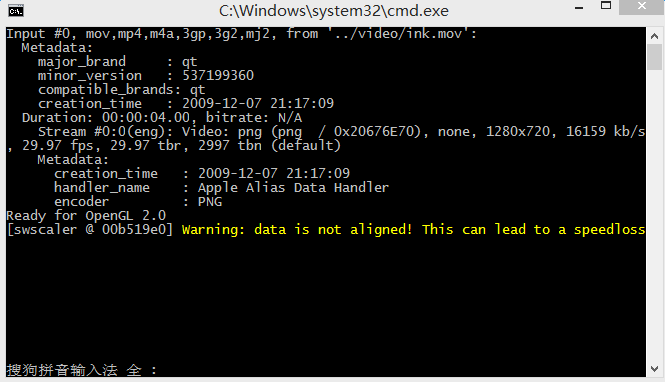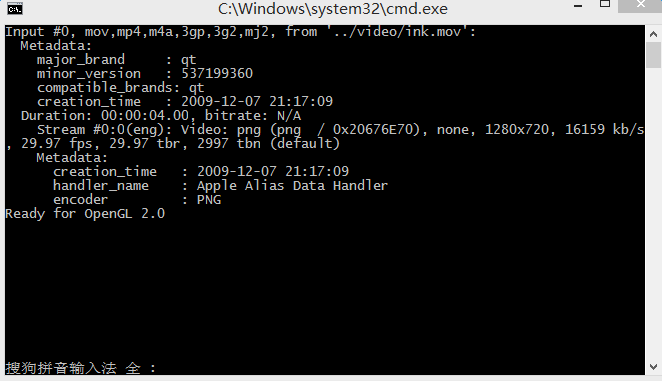# [swscaler] Warning: data is not aligned! This can lead to a speedloss 的解决方法【FFmpeg】

## [swscaler] Warning: data is not aligned! This can lead to a speedloss 的解决m_dst_h = (dst_h >> 4) << 4 ;
m_dst_w = (dst_w >> 4) <<4 ;

	float ratio = 1.0f * getSrcWidth()/getSrcHeight();
m_dst_h = (dst_h >> 4) << 4 ;
m_dst_w = (int(ratio * m_dst_h)>>4)  <<4 ;size = (size + 0xf) & ~0xf;

 int tmp = 1;
int w = bitmap.getWidth();
int h = bitmap.getHeight();
while (w > tmp || h > tmp) {
tmp <<= 1;
}

int width = tmp;
int height = tmp;

©️2019 CSDN 皮肤主题: 1024 设计师: 上身试试